# Decision Trees, Forests, and Nearest-Neighbors classifiers

The classic statistical decision theory on which LDA and QDA, and logistic regression are highly model-based. We assume the features are fit by some model, we fit that model, and use inferences from that model to make a decision. Using the model means we make assumptions, and if those assumptions are correct, we can have a lot of success. Not all classifiers make such strong assumptions, and three of these will be covered in this section: Decision trees, random forests, and K-Nearest Neighbor classifiers.

# Decision trees

Decision trees assume that the different predictors are independent and combine together to form a an overall likelihood of one class over another. However, this may not be true. Many times, we might want to make a classification rule based on a few simple measures. The notion is that you may have several measures, and by asking a few decisions about individual dimensions, end up with a classification decision. For example, such trees are frequently used in medical contexts. If you want to know if you are at risk for some disease, the decision process might be a series of yes/no questions, at the end of which a ‘high-risk’ or ‘low-risk’ label would be given:

1. Decision 1: Are you above 35?
• Yes: Use Decision 2
• No: Use Decision 3
1. Decision 2: Do you have testing scores above 1000?
• Yes: High risk
• No: Low risk
1. Decision 3: Do you a family history?
• Yes: etc.
• No: etc.

Notice that if we were trying to determine a class via LDA, we’d create a single composite score based on all the questions and make a decision at the end. This allows us to consider interactions between features conditionally, and so it can be very powerful. Tree-based decision tools can be useful in many cases:

• When we want simple decision rules that can be applied by novices or people under time stress.
• When the structure of our classes are dependent or nested, or somehow hierarchical. Many natural categories have a hierarchical structure, so that the way you split one variable may depend on the value of another. For example, if you want to know if someone is ‘tall’, you first might determine their gender, and then use a different cutoff for each gender.
• When many of our observables are binary states. To classify lawmakers we can look at their voting patterns–which are always binary. We may be able to identify just a couple issues we care about that will tell us everything we need to know.
• When you have multiple categories. There is no need to restrict the classes to binary, unlike for the previous methods we examined.

To make a decision tree, we essentially have to use heuristic processes to determine the best order and cutoffs to use in making decisions, while identifying our error rates. Even with a single feature, we can make a decision tree that correctly classifies all the elements in a set (as long as all feature values are unique). So we also need to understand how many rules to use, in order to minimize over-fitting.

There are a number of software packages available for classification trees. One commonly-used package in R is called rpart.

’Let’s start with a simple tree made to classify elements on which we have only one measure:

library(rpart)
library(rattle)  #for graphing
library(rpart.plot)  #also for graphing
library(DAAG)
classes <- sample(c("A", "B"), 100, replace = T)
predictor <- rnorm(100)
r1 <- rpart(classes ~ predictor, method = "class")

plot(r1)
text(r1)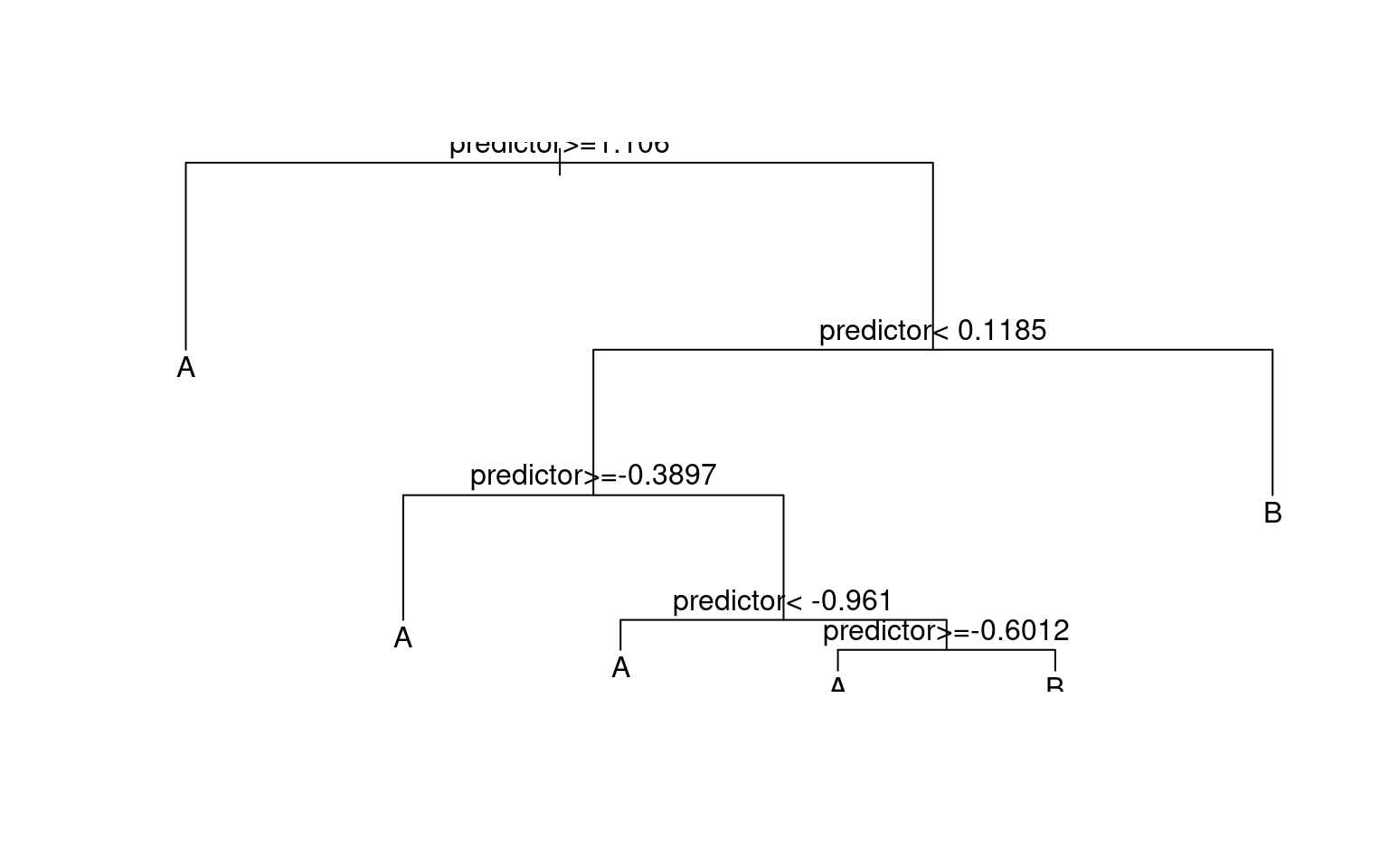prp(r1)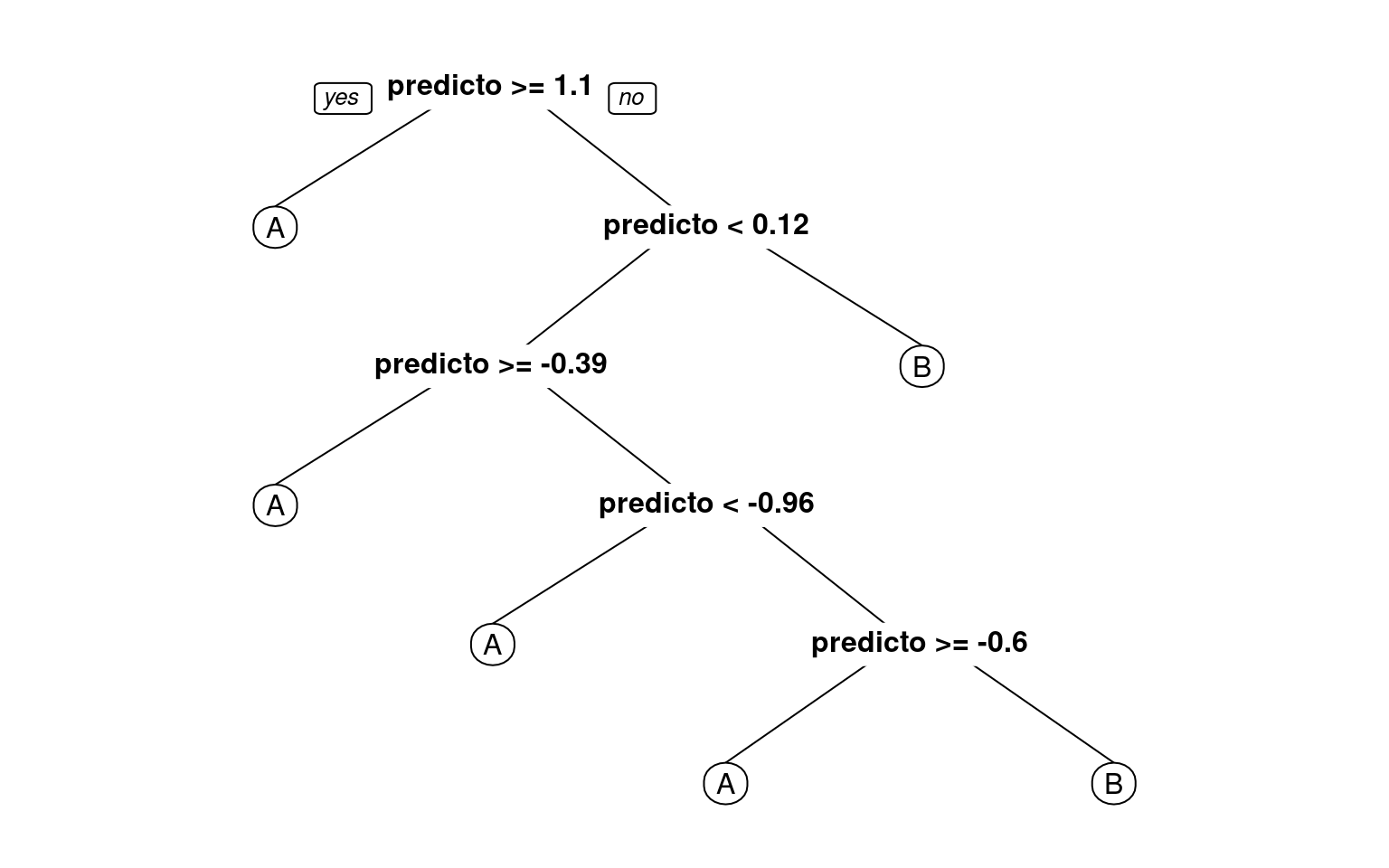fancyRpartPlot(r1)  #better visualization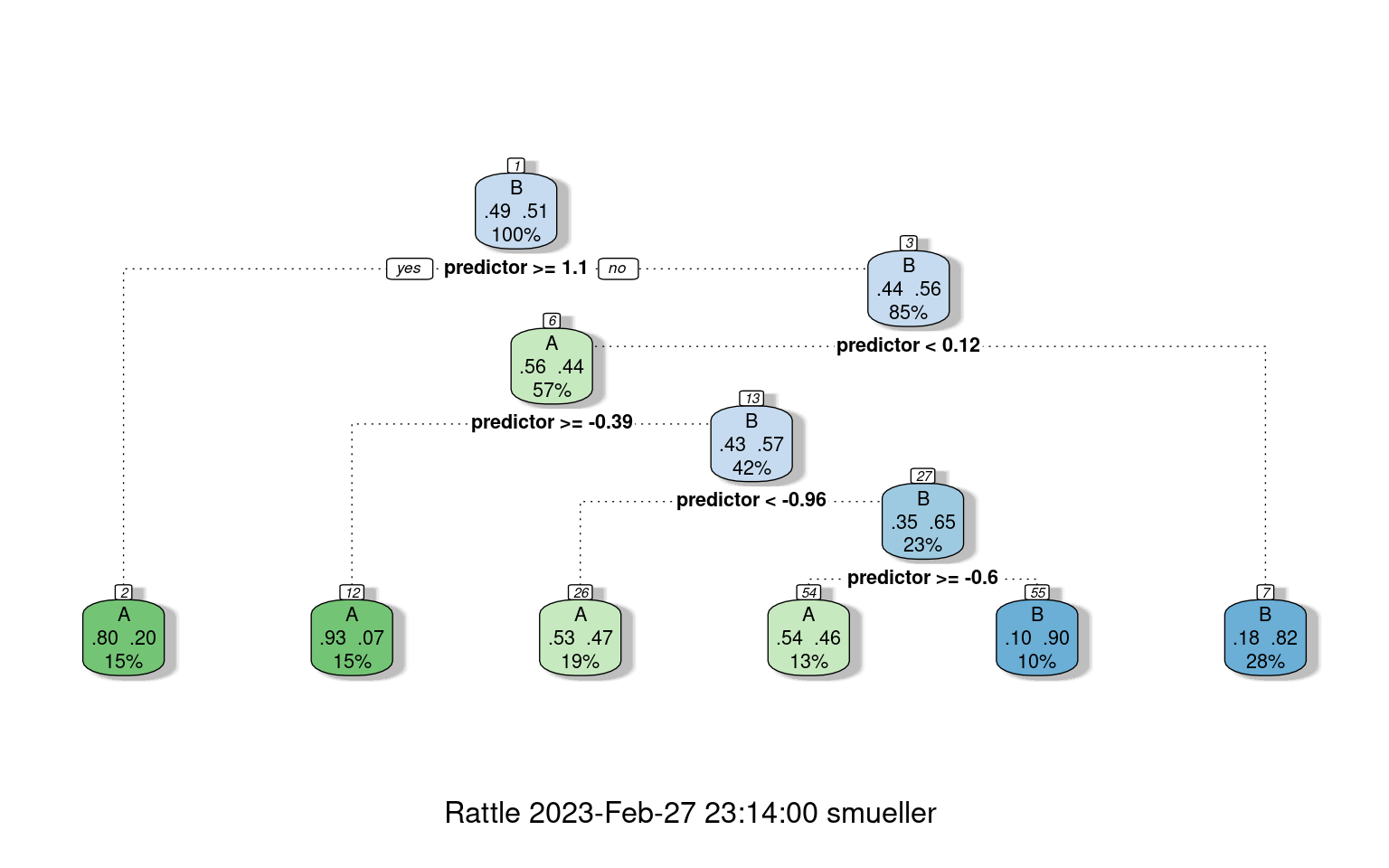confusion(classes, predict(r1, type = "class"))
Overall accuracy = 0.68

Confusion matrix
Predicted (cv)
Actual  [,1]  [,2]
[1,] 0.619 0.381
[2,] 0.276 0.724

BNotice that even though the predictor had no true relationship to the categories, we were able to get 71% accuracy. We could in fact do better, just by adding rules:

r2 <- rpart(classes ~ predictor, method = "class", control = c(minsplit = 1, minbucket = 1,
cp = -1))
prp(r2)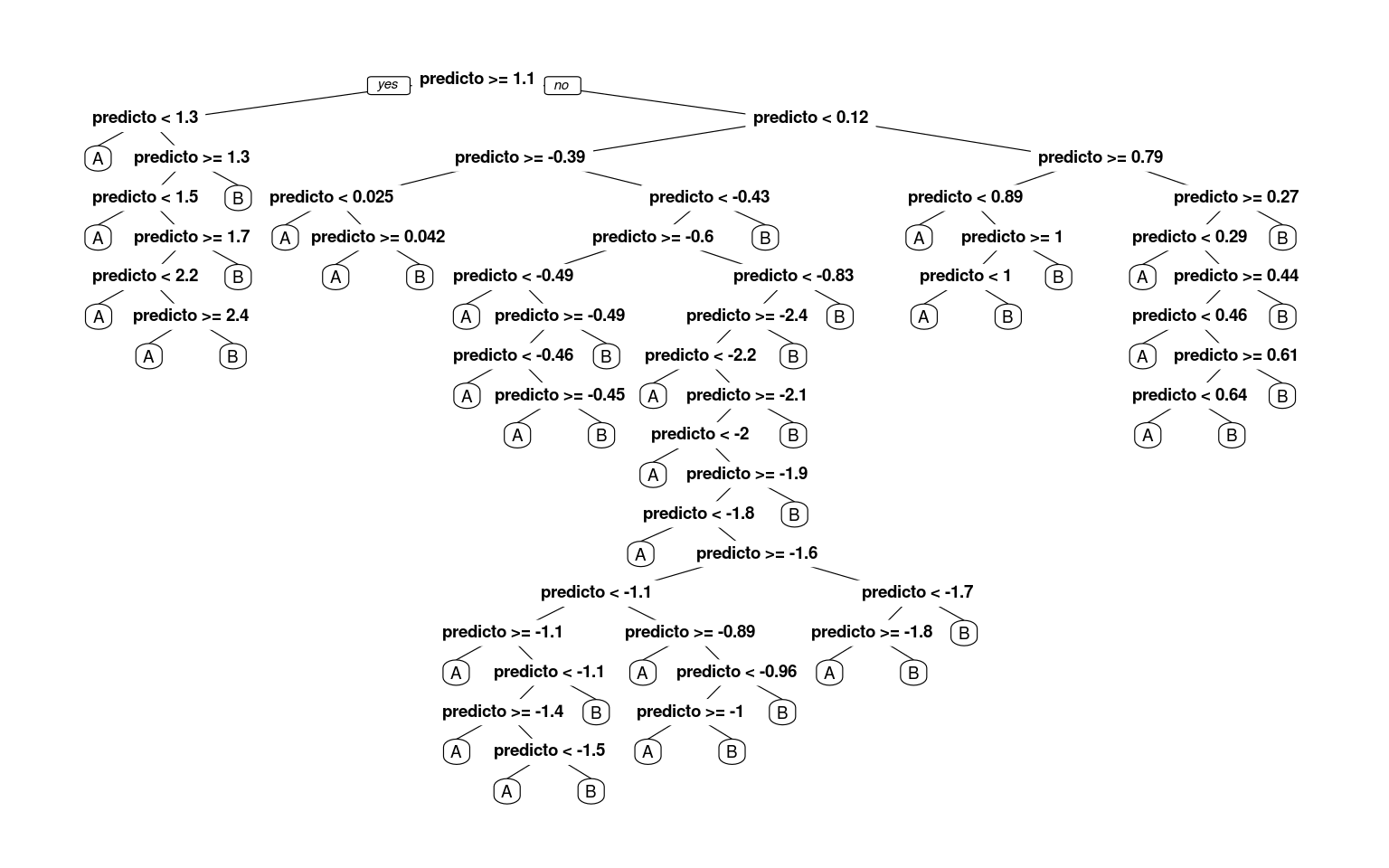# this tree is a bit too large for this graphics method: fancyRpartPlot(r2)
confusion(classes, predict(r2, type = "class"))
Overall accuracy = 1

Confusion matrix
Predicted (cv)
Actual [,1] [,2]
[1,]    1    0
[2,]    0    1

Now, we have completely predicted every item by successively dividing the line. There were three parameters we adjusted to do this. First, we set the minsplit and minbucket to 1–this determines the smallest size of the leaf nodes. Then, we set the cp argument to be negative (it defaults to .01). cp is a complexity parameter, that is a criterion for how much better each model must be before splitting a leaf node. A negative value will mean that any additional node is better. Notice that by default, rpart must choose the best number of nodes to use. This is a critical decision for a decision tree, as it impacts the complexity of the model, and how much it overfits the data.

How will this work for a real data set? Let’s re-load the engineering data set. In this data, we have asked both Engineering and Psychology students to determine whether pairs of words from Psychology, Engineering go together, and measured their time and accuracy.

joint <- read.csv("eng-joint.csv")
joint$eng <- as.factor(c("psych", "eng")[joint$eng + 1])

## This is the partitioning tree:

r1 <- rpart(eng ~ ., data = joint, method = "class")
r1
n= 76

node), split, n, loss, yval, (yprob)
* denotes terminal node

1) root 76 38 eng (0.50000000 0.50000000)
2) ep.1>=2049.256 68 31 eng (0.54411765 0.45588235)
4) ppr.1< 2900.149 39 14 eng (0.64102564 0.35897436)
8) ppr.1>=2656.496 11  1 eng (0.90909091 0.09090909) *
9) ppr.1< 2656.496 28 13 eng (0.53571429 0.46428571)
18) ep>=0.7083333 14  4 eng (0.71428571 0.28571429) *
19) ep< 0.7083333 14  5 psych (0.35714286 0.64285714) *
5) ppr.1>=2900.149 29 12 psych (0.41379310 0.58620690)
10) ppu>=0.8333333 7  2 eng (0.71428571 0.28571429) *
11) ppu< 0.8333333 22  7 psych (0.31818182 0.68181818) *
3) ep.1< 2049.256 8  1 psych (0.12500000 0.87500000) *
prp(r1, cex = 0.75)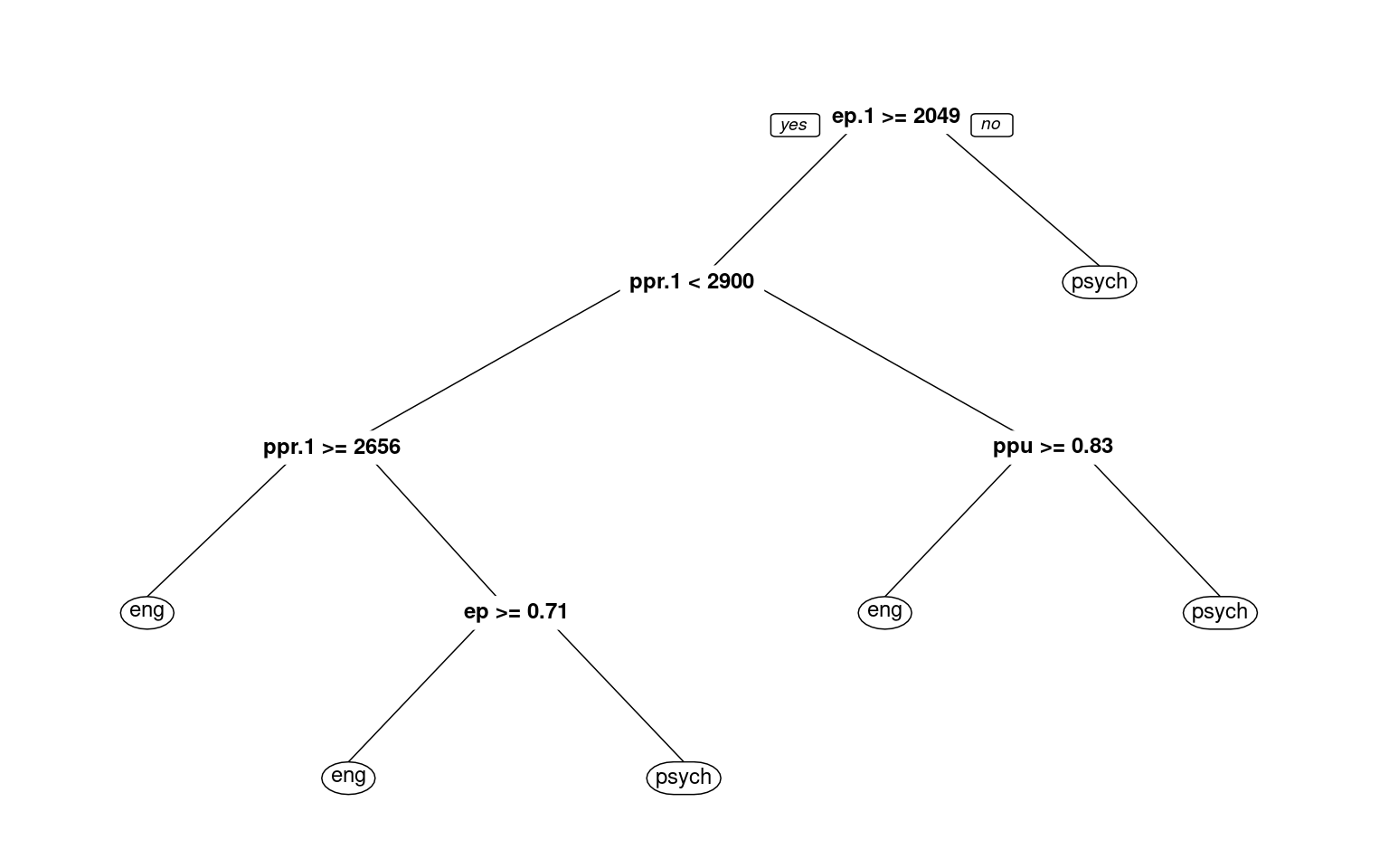# text(r1,use.n=TRUE)
fancyRpartPlot(r1)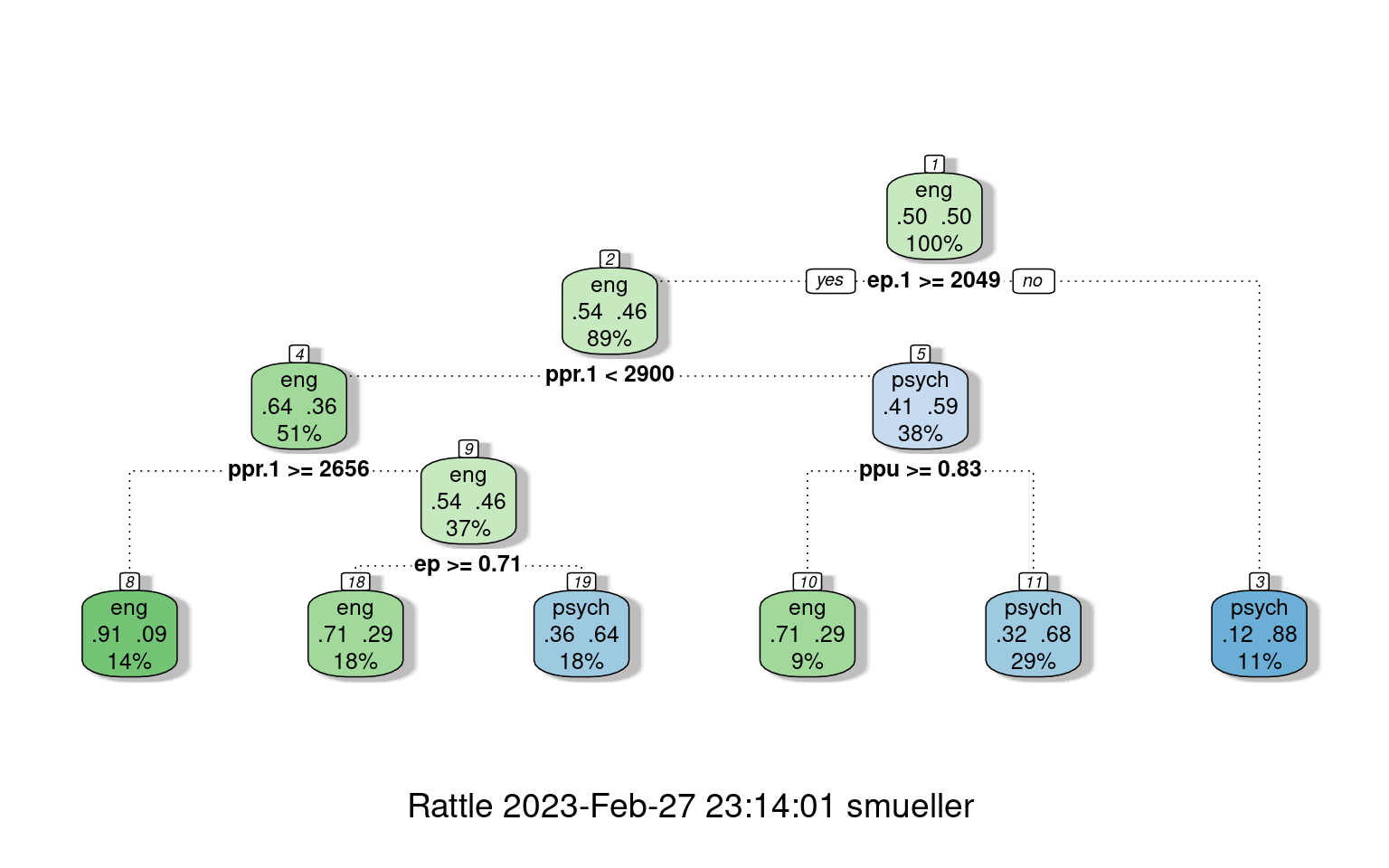confusion(joint$eng, predict(r1, type = "class")) Overall accuracy = 0.737 Confusion matrix Predicted (cv) Actual eng psych eng 0.658 0.342 psych 0.184 0.816 With the default full partitioning, we get 73% accuracy.. But the decision tree is fairly complicated, and we might want something simpler. Let’s only allow it to go to a depth of 2, by controlling maxdepth: r2 <- rpart(eng ~ ., data = joint, method = "class", control = c(maxdepth = 3)) r2 n= 76 node), split, n, loss, yval, (yprob) * denotes terminal node 1) root 76 38 eng (0.5000000 0.5000000) 2) ep.1>=2049.256 68 31 eng (0.5441176 0.4558824) 4) ppr.1< 2900.149 39 14 eng (0.6410256 0.3589744) * 5) ppr.1>=2900.149 29 12 psych (0.4137931 0.5862069) 10) ppu>=0.8333333 7 2 eng (0.7142857 0.2857143) * 11) ppu< 0.8333333 22 7 psych (0.3181818 0.6818182) * 3) ep.1< 2049.256 8 1 psych (0.1250000 0.8750000) * fancyRpartPlot(r2) text(r2, use.n = T)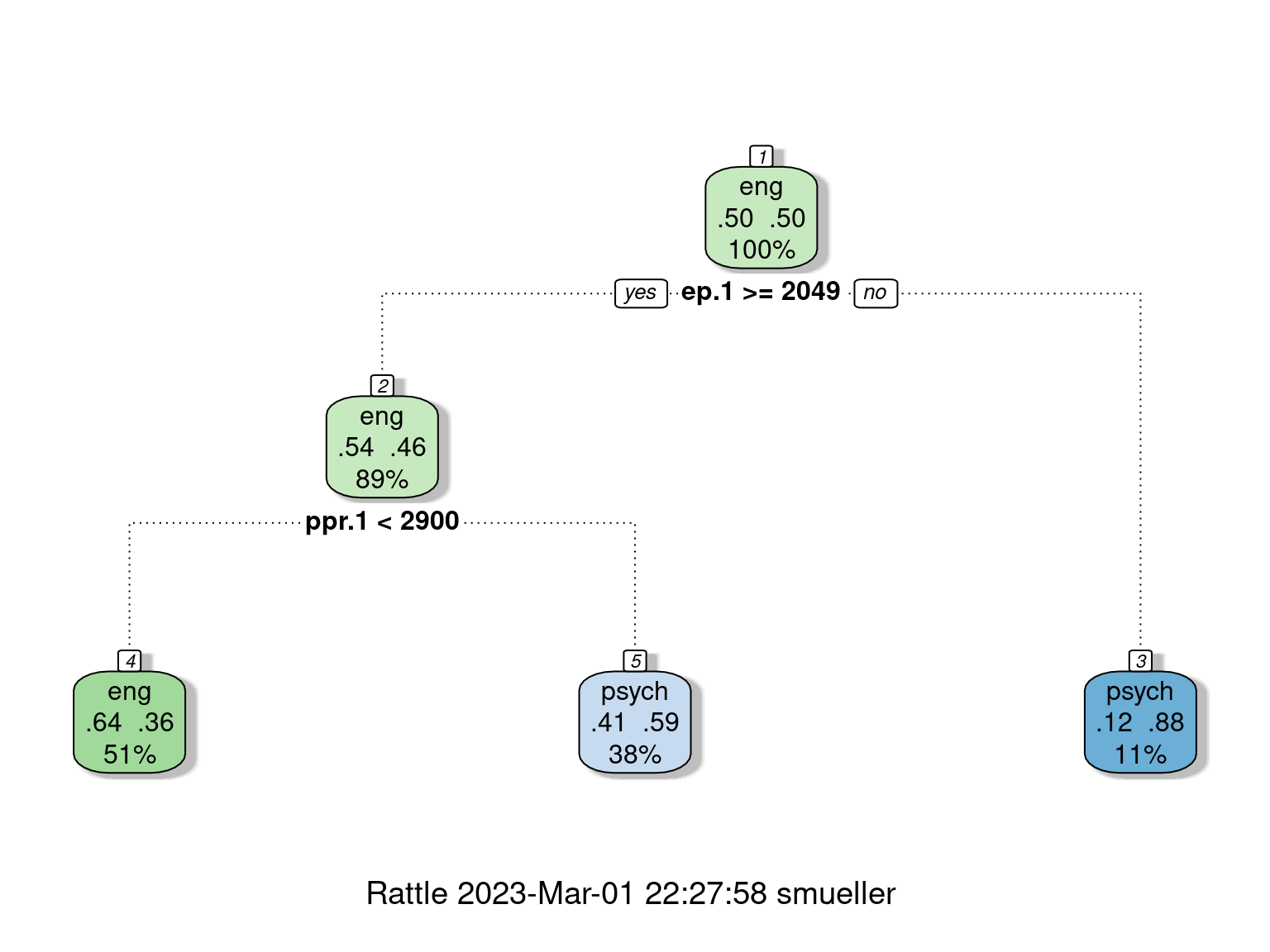confusion(joint$eng, predict(r2, type = "class"))
Overall accuracy = 0.684

Confusion matrix
Predicted (cv)
Actual    eng psych
eng   0.789 0.211
psych 0.421 0.579

Here, we are down to 68% ‘correct’ classifications.

## Looking deeper

If we look at the summary of the tree object, it gives us a lot of details about goodness of fit and decision points.

summary(r2)
Call:
rpart(formula = eng ~ ., data = joint, method = "class", control = c(maxdepth = 3))
n= 76

CP nsplit rel error   xerror      xstd
1 0.15789474      0 1.0000000 1.263158 0.1106647
2 0.13157895      1 0.8421053 1.289474 0.1097968
3 0.07894737      2 0.7105263 1.236842 0.1114442
4 0.01000000      3 0.6315789 1.184211 0.1127448

Variable importance
ep.1 ppr.1 ppu.1   ppu eer.1 eeu.1    ep
25    19    16    14    13    11     1

Node number 1: 76 observations,    complexity param=0.1578947
predicted class=eng    expected loss=0.5  P(node) =1
class counts:    38    38
probabilities: 0.500 0.500
left son=2 (68 obs) right son=3 (8 obs)
Primary splits:
ep.1  < 2049.256  to the right, improve=2.514706, (0 missing)
eeu.1 < 2431.336  to the right, improve=2.326531, (0 missing)
ppu.1 < 4579.384  to the right, improve=2.072727, (0 missing)
eer.1 < 4687.518  to the right, improve=1.966874, (0 missing)
ppu   < 0.8333333 to the right, improve=1.719298, (0 missing)
Surrogate splits:
ppu.1 < 1637.18   to the right, agree=0.947, adj=0.500, (0 split)
eeu.1 < 1747.891  to the right, agree=0.934, adj=0.375, (0 split)
eer.1 < 1450.41   to the right, agree=0.921, adj=0.250, (0 split)
ppr.1 < 1867.892  to the right, agree=0.921, adj=0.250, (0 split)

Node number 2: 68 observations,    complexity param=0.1315789
predicted class=eng    expected loss=0.4558824  P(node) =0.8947368
class counts:    37    31
probabilities: 0.544 0.456
left son=4 (39 obs) right son=5 (29 obs)
Primary splits:
ppr.1 < 2900.149  to the left,  improve=1.717611, (0 missing)
ppu   < 0.8333333 to the right, improve=1.553072, (0 missing)
ppu.1 < 4579.384  to the right, improve=1.535294, (0 missing)
eer.1 < 4687.518  to the right, improve=1.529205, (0 missing)
ep.1  < 2754.762  to the left,  improve=1.013682, (0 missing)
Surrogate splits:
eer.1 < 3007.036  to the left,  agree=0.750, adj=0.414, (0 split)
ep.1  < 2684.733  to the left,  agree=0.647, adj=0.172, (0 split)
ppu.1 < 2346.981  to the left,  agree=0.632, adj=0.138, (0 split)
eeu.1 < 2806.34   to the left,  agree=0.618, adj=0.103, (0 split)
ep    < 0.7916667 to the left,  agree=0.603, adj=0.069, (0 split)

Node number 3: 8 observations
predicted class=psych  expected loss=0.125  P(node) =0.1052632
class counts:     1     7
probabilities: 0.125 0.875

Node number 4: 39 observations
predicted class=eng    expected loss=0.3589744  P(node) =0.5131579
class counts:    25    14
probabilities: 0.641 0.359

Node number 5: 29 observations,    complexity param=0.07894737
predicted class=psych  expected loss=0.4137931  P(node) =0.3815789
class counts:    12    17
probabilities: 0.414 0.586
left son=10 (7 obs) right son=11 (22 obs)
Primary splits:
ppu   < 0.8333333 to the right, improve=1.6663680, (0 missing)
ppr.1 < 4858.184  to the right, improve=1.6663680, (0 missing)
eeu.1 < 3128.98   to the right, improve=1.5785810, (0 missing)
ep    < 0.625     to the left,  improve=1.0584390, (0 missing)
eer.1 < 3323.144  to the right, improve=0.8880131, (0 missing)
Surrogate splits:
ppu.1 < 6007.29   to the right, agree=0.828, adj=0.286, (0 split)
eer.1 < 4516.42   to the right, agree=0.793, adj=0.143, (0 split)
eeu.1 < 3768.95   to the right, agree=0.793, adj=0.143, (0 split)
ep.1  < 5327.593  to the right, agree=0.793, adj=0.143, (0 split)

Node number 10: 7 observations
predicted class=eng    expected loss=0.2857143  P(node) =0.09210526
class counts:     5     2
probabilities: 0.714 0.286

Node number 11: 22 observations
predicted class=psych  expected loss=0.3181818  P(node) =0.2894737
class counts:     7    15
probabilities: 0.318 0.682 

This model seems to fit a lot better than our earlier LDA models, which suggest that it is probably overfitting. Cross-validation can be done within the control parameter $$xval$$:

r3 <- rpart(eng ~ ., data = joint, method = "class", control = c(maxdepth = 3, xval = 10))
r3
n= 76

node), split, n, loss, yval, (yprob)
* denotes terminal node

1) root 76 38 eng (0.5000000 0.5000000)
2) ep.1>=2049.256 68 31 eng (0.5441176 0.4558824)
4) ppr.1< 2900.149 39 14 eng (0.6410256 0.3589744) *
5) ppr.1>=2900.149 29 12 psych (0.4137931 0.5862069)
10) ppu>=0.8333333 7  2 eng (0.7142857 0.2857143) *
11) ppu< 0.8333333 22  7 psych (0.3181818 0.6818182) *
3) ep.1< 2049.256 8  1 psych (0.1250000 0.8750000) *
confusion(joint$eng, predict(r1, type = "class")) Overall accuracy = 0.737 Confusion matrix Predicted (cv) Actual eng psych eng 0.658 0.342 psych 0.184 0.816 confusion(joint$eng, predict(r3, type = "class"))
Overall accuracy = 0.684

Confusion matrix
Predicted (cv)
Actual    eng psych
eng   0.789 0.211
psych 0.421 0.579

Accuracy goes down a bit, but the 74% accuracy is about what we achieved in the simple lda models.

Clearly, for partitioning trees, we have to be careful about overfitting, because we can always easily get the perfect classification.

## Regression Trees

Instead of using a tree to divide and predict group memberships, rpart can also use a tree as a sort of regression. It tries to model all of the data within a group as a single intercept value, and then tries to divide groups to improve fit. There are some pdf help files available for more detail, but the regression options (including poisson and anova) are a bit poorly documented. But, basically, we can use the same ideas to partition the data, and then fit either a single value within each group or some small linear model. The tree shows nodes with the the top value the mean for that branch.

cw <- rpart(weight ~ Time + Diet, data = ChickWeight, control = rpart.control(maxdepth = 5))
summary(cw)
Call:
rpart(formula = weight ~ Time + Diet, data = ChickWeight, control = rpart.control(maxdepth = 5))
n= 578

CP nsplit rel error    xerror       xstd
1 0.54964983      0 1.0000000 1.0036709 0.06390682
2 0.08477800      1 0.4503502 0.4706970 0.03591395
3 0.04291979      2 0.3655722 0.3770360 0.02952526
4 0.03905827      3 0.3226524 0.3449287 0.02888378
5 0.01431308      4 0.2835941 0.3184773 0.02699598
6 0.01000000      5 0.2692810 0.3118980 0.02692255

Variable importance
Time Diet
93    7

Node number 1: 578 observations,    complexity param=0.5496498
mean=121.8183, MSE=5042.484
left son=2 (296 obs) right son=3 (282 obs)
Primary splits:
Time < 11 to the left,  improve=0.54964980, (0 missing)
Diet splits as  LRRR,   improve=0.04479917, (0 missing)

Node number 2: 296 observations,    complexity param=0.04291979
mean=70.43243, MSE=700.6779
left son=4 (149 obs) right son=5 (147 obs)
Primary splits:
Time < 5  to the left,  improve=0.60314240, (0 missing)
Diet splits as  LRRR,   improve=0.04085943, (0 missing)

Node number 3: 282 observations,    complexity param=0.084778
mean=175.7553, MSE=3919.043
left son=6 (144 obs) right son=7 (138 obs)
Primary splits:
Time < 17 to the left,  improve=0.2235767, (0 missing)
Diet splits as  LLRR,   improve=0.1333256, (0 missing)

Node number 4: 149 observations
mean=50.01342, MSE=71.0468

Node number 5: 147 observations
mean=91.12925, MSE=487.9085

Node number 6: 144 observations,    complexity param=0.01431308
mean=146.7778, MSE=1825.326
left son=12 (84 obs) right son=13 (60 obs)
Primary splits:
Diet splits as  LLRR,   improve=0.1587094, (0 missing)
Time < 15 to the left,  improve=0.1205157, (0 missing)

Node number 7: 138 observations,    complexity param=0.03905827
mean=205.9928, MSE=4313.283
left son=14 (80 obs) right son=15 (58 obs)
Primary splits:
Diet splits as  LLRR,   improve=0.19124870, (0 missing)
Time < 19 to the left,  improve=0.02989732, (0 missing)

Node number 12: 84 observations
mean=132.3929, MSE=1862.834

Node number 13: 60 observations
mean=166.9167, MSE=1077.543

Node number 14: 80 observations
mean=181.5375, MSE=3790.849

Node number 15: 58 observations
mean=239.7241, MSE=3071.165 
fancyRpartPlot(cw)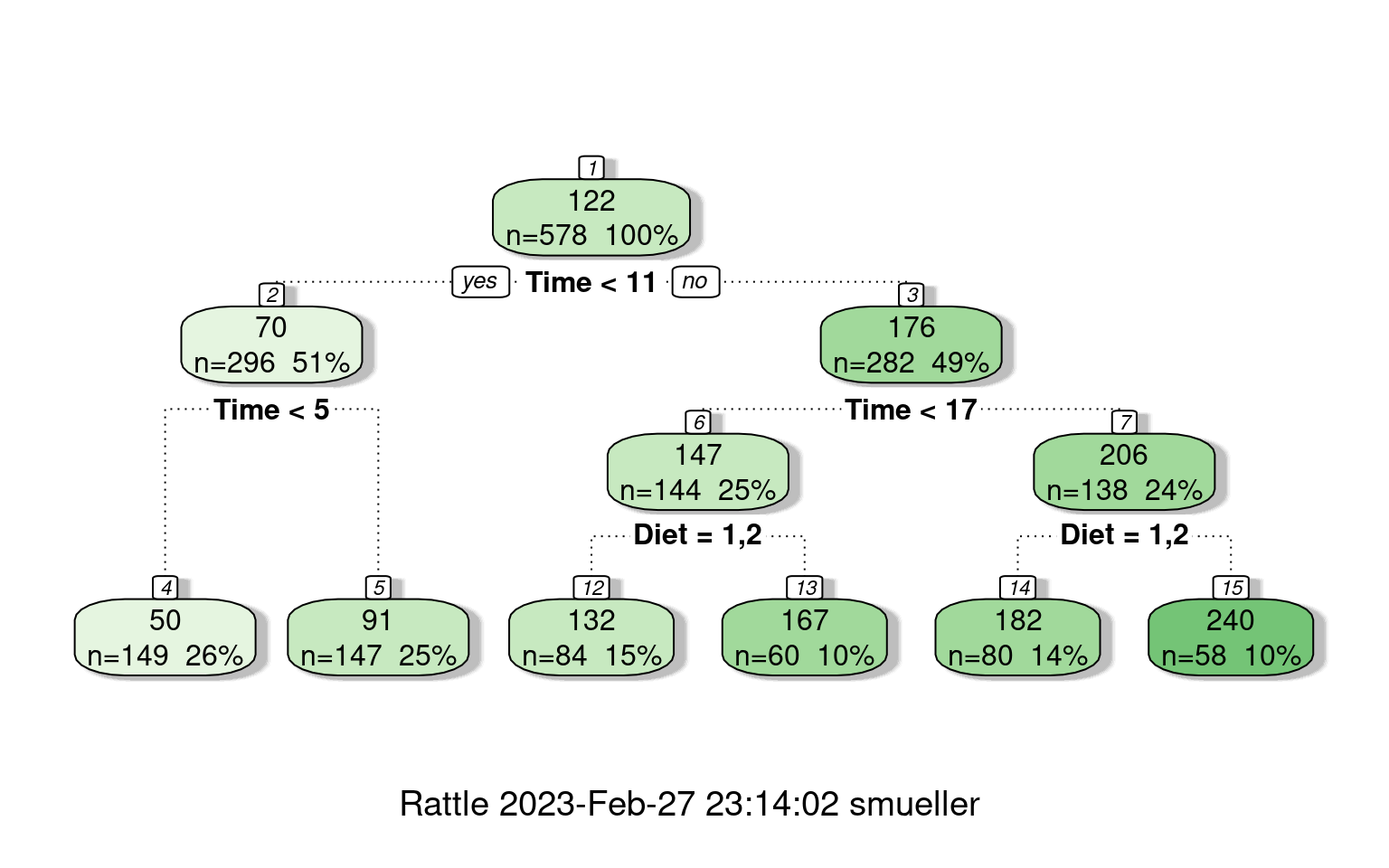## this shows the yvalue estimate for each leaf node:
cw$frame  var n wt dev yval complexity ncompete nsurrogate 1 Time 578 578 2914555.93 121.81834 0.549649827 1 0 2 Time 296 296 207400.65 70.43243 0.042919791 1 0 4 <leaf> 149 149 10585.97 50.01342 0.010000000 0 0 5 <leaf> 147 147 71722.54 91.12925 0.007137211 0 0 3 Time 282 282 1105170.12 175.75532 0.084778005 1 0 6 Diet 144 144 262846.89 146.77778 0.014313079 1 0 12 <leaf> 84 84 156478.04 132.39286 0.005288100 0 0 13 <leaf> 60 60 64652.58 166.91667 0.005342978 0 0 7 Diet 138 138 595232.99 205.99275 0.039058272 1 0 [ reached 'max' / getOption("max.print") -- omitted 2 rows ] library(ggplot2) ChickWeight$predicted <- predict(cw)
ggplot(ChickWeight, aes(x = Time, y = predicted, group = Diet, color = Diet, size = Diet)) +
geom_point(shape = 1)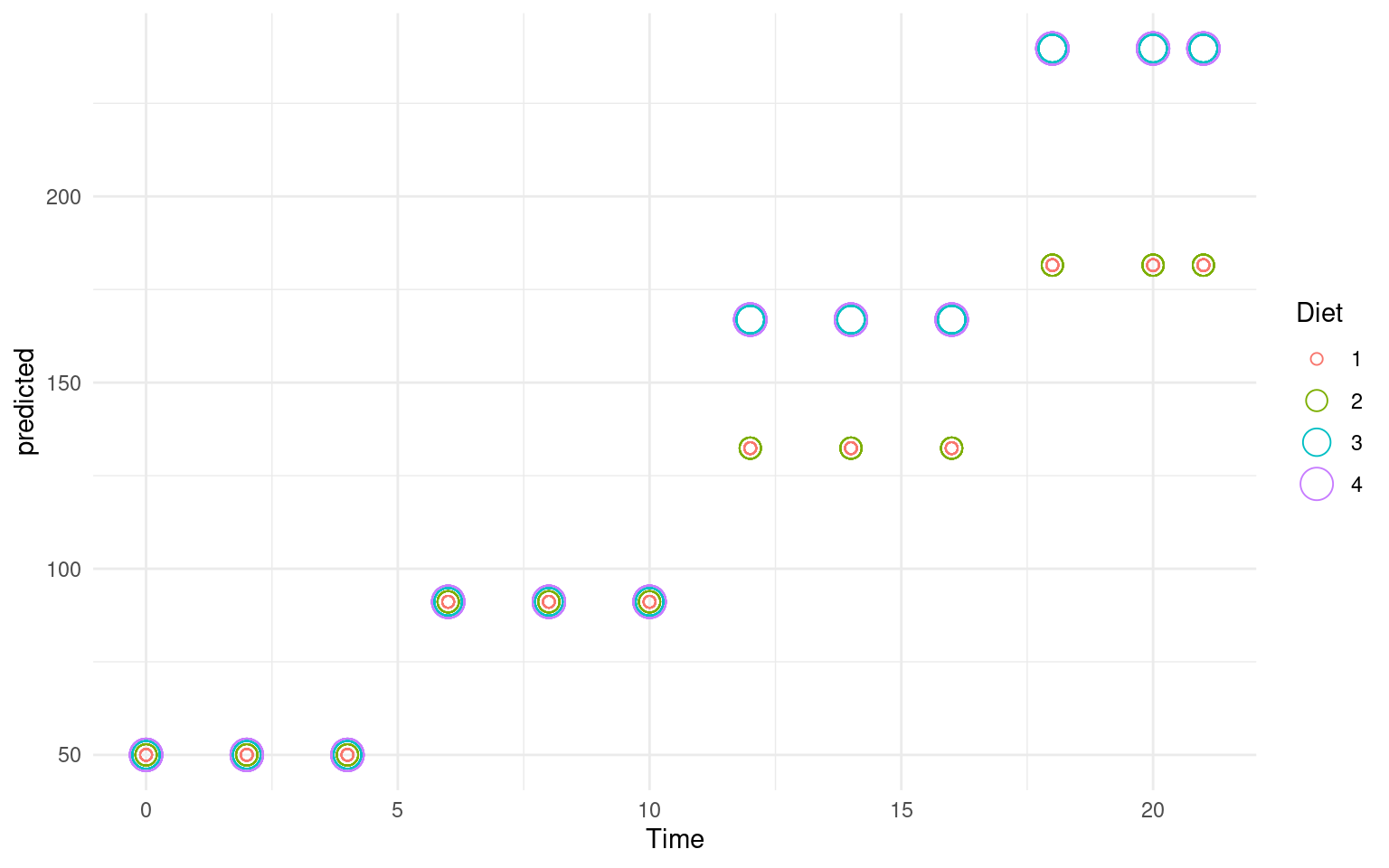# Random Forests

One advantage of partitioning/decision trees is that they are fast and easy to make. They are also considered interpretable and easy to understand. A tree like this can be used by a doctor or a medical counselor to help understand the risk for a disease, by asking a few simple questions.

The downsides of a decision tree is that they are often not very good, especially once they have been trimmed to avoid over-fitting. Recently, researchers have been interested in combining the results of many small (and often not-very-good) classifiers to make one better one. This often is described as ‘boosting’, or ensemble’ methods, and there are a number of ways to achieve this. Doing this in a particular way with decision trees is referred to as a ‘random forest’ (see Breiman and Cutler).

Random forests can be used for both regression and classification (trees can be used in either way as well), and the classification and regression trees (CART) approach is a method that supports both. A random forest works as follows:

• Build $$N$$ trees (where N may be hundreds), where each tree is built from a random subset of features/variables. That is, on each step, choose the best variable to divide the branch based on a random subset of variables.

For each tree:

1. Pick a subset of the variables.
2. Build a tree
• Then, to classify any item, have each tree determine its best guess, and then take the most frequent outcome (or give a probabilistic answer based on the balance of evidence).

The randomForest package in R supports building these models. Because these can be a bit more difficult to comprehend, there is a companion package randomForestExplainer that is also handy in digging down on the types of forests derived.

library(randomForest)
library(randomForestExplainer)

rf <- randomForest(x = joint[, -1], y = joint$eng, proximity = T, ntree = 5) rf  Call: randomForest(x = joint[, -1], y = joint$eng, ntree = 5, proximity = T)
Type of random forest: classification
Number of trees: 5
No. of variables tried at each split: 3

OOB estimate of  error rate: 51.52%
Confusion matrix:
eng psych class.error
eng    13    20   0.6060606
psych  14    19   0.4242424
# printRandomForests(rf) ##look at all the RFs
confusion(joint$eng, predict(rf)) Overall accuracy = 0.485 Confusion matrix Predicted (cv) Actual eng psych eng 0.394 0.606 psych 0.424 0.576 If you run this repeatedly, you get a different answer each time. For this data, quite often the accuracy is below chance. The confusion matrix produced is “OOB”: out-of-bag, which is like Leave-on-out cross-validation. Looking at the trees, they are quite complex as well, with tens of rules. We can get a printout of individual subtrees with getTree: (getTree(rf, 1))  left daughter right daughter split var split point status prediction 1 2 3 4 0.5000000 1 0 2 4 5 10 4508.9876666 1 0 3 6 7 3 0.7916667 1 0 4 8 9 9 3215.1130121 1 0 5 10 11 10 5213.0938222 1 0 6 12 13 9 2886.5132720 1 0 7 14 15 9 4314.5655096 1 0 8 16 17 9 1678.8421774 1 0 9 18 19 2 0.5000000 1 0 10 0 0 0 0.0000000 -1 1 11 0 0 0 0.0000000 -1 2 12 20 21 3 0.6250000 1 0 [ reached getOption("max.print") -- omitted 31 rows ] (getTree(rf, 5))  left daughter right daughter split var split point status prediction 1 2 3 3 0.4583333 1 0 2 0 0 0 0.0000000 -1 1 3 4 5 6 2742.3646520 1 0 4 6 7 2 0.5000000 1 0 5 8 9 5 0.1666667 1 0 6 10 11 6 2640.9514337 1 0 7 12 13 10 1532.3915974 1 0 8 0 0 0 0.0000000 -1 1 9 14 15 8 6748.1246942 1 0 10 0 0 0 0.0000000 -1 2 11 0 0 0 0.0000000 -1 1 12 0 0 0 0.0000000 -1 2 [ reached getOption("max.print") -- omitted 27 rows ] There are many arguments that support the sampling/evaluation process. The feasibility of changing these will often depend on the size of the data set. With a large data set and many variables, fitting one of these models may take a long time if they are not constrained. Here we look at: • replace: cases are sampled with or without replacement • mtry: how many variables to try at each split • ntree: how many trees to grow • keep.inbag: keep track of which samples are ‘in the bag’ • sampsize: number of samples to try. rf <- randomForest(eng ~ ., data = joint, proximity = T, replace = F, mtry = 3, ntree = 50, keep.inbag = T, sampsize = 10, localImp = TRUE) confusion(joint$eng, predict(rf))
Overall accuracy = 0.368

Confusion matrix
Predicted (cv)
Actual    eng psych
eng   0.421 0.579
psych 0.684 0.316

The forest lets us understand which variables are more important, by identifying how often variables are closer to the root of the tree. Depending on the run, these figures change, but a variable that is frequently selected as the root, or one whose mean depth is low, is likely to be more important.

plot_min_depth_distribution(rf)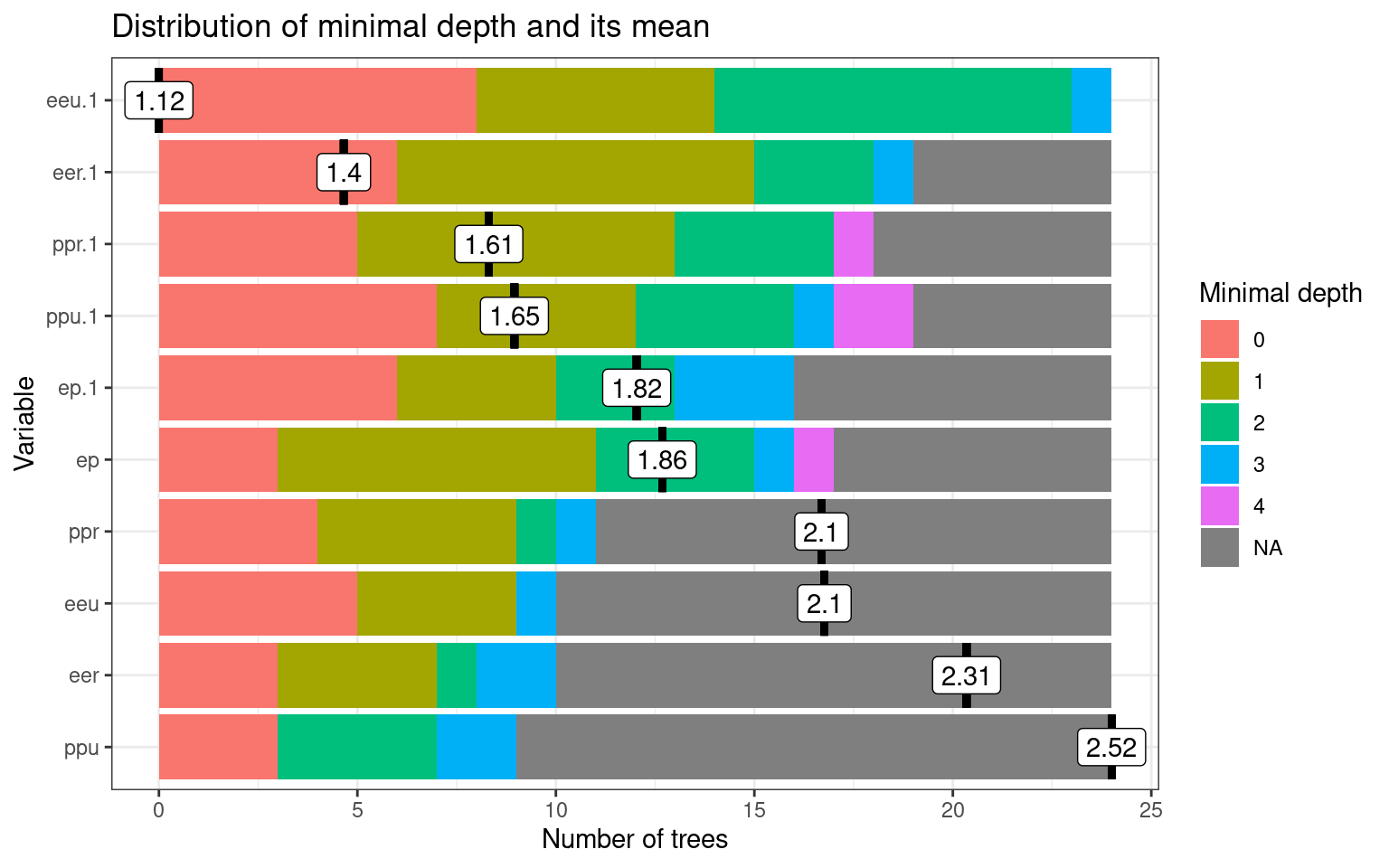plot_multi_way_importance(rf)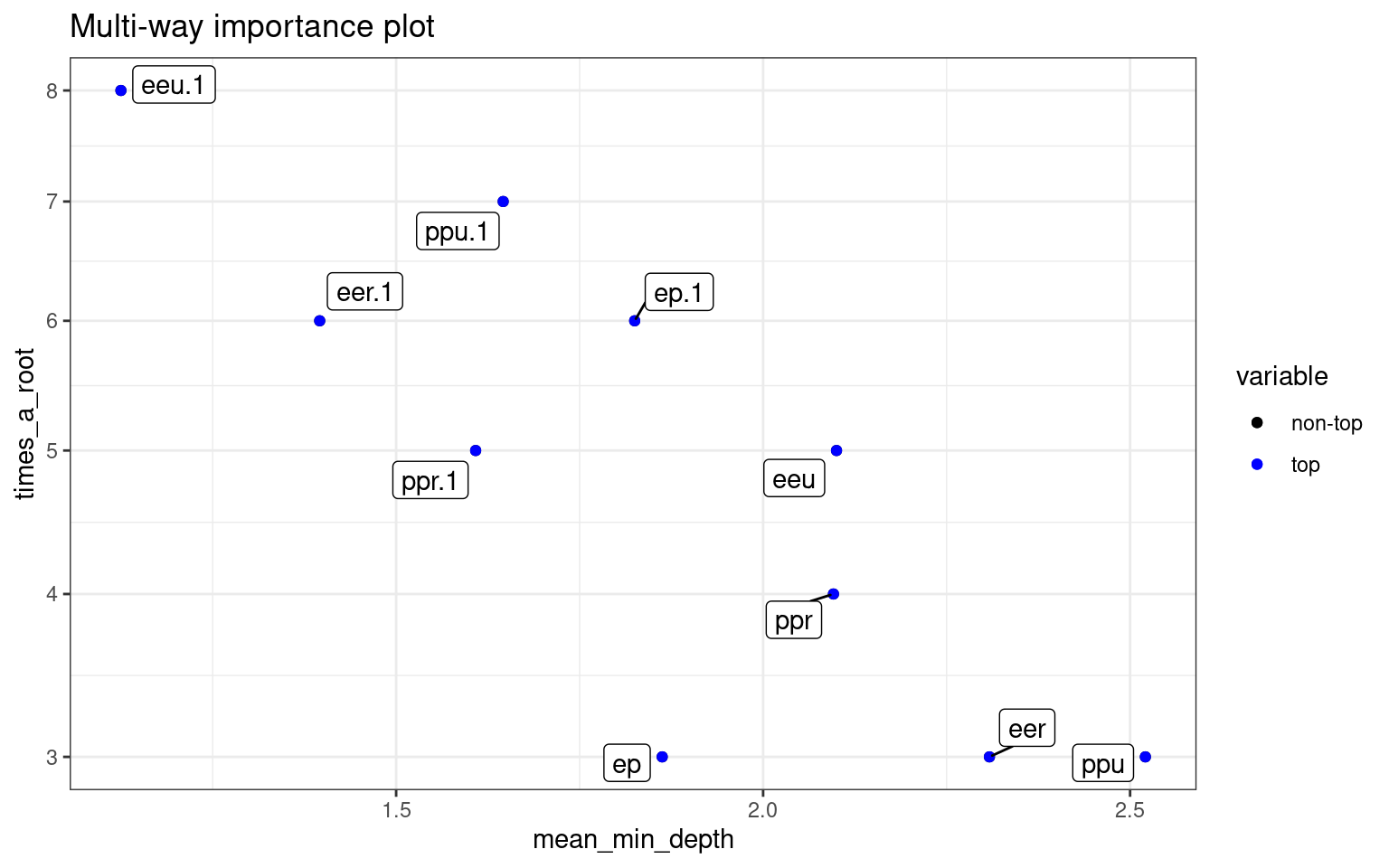This plots the prediction of the forest for different values of variables. If the variables are useful, we will tend to see blocks of red/purple indicating the prediction in different regions.:

plot_predict_interaction(rf, joint, "eer", "ppr")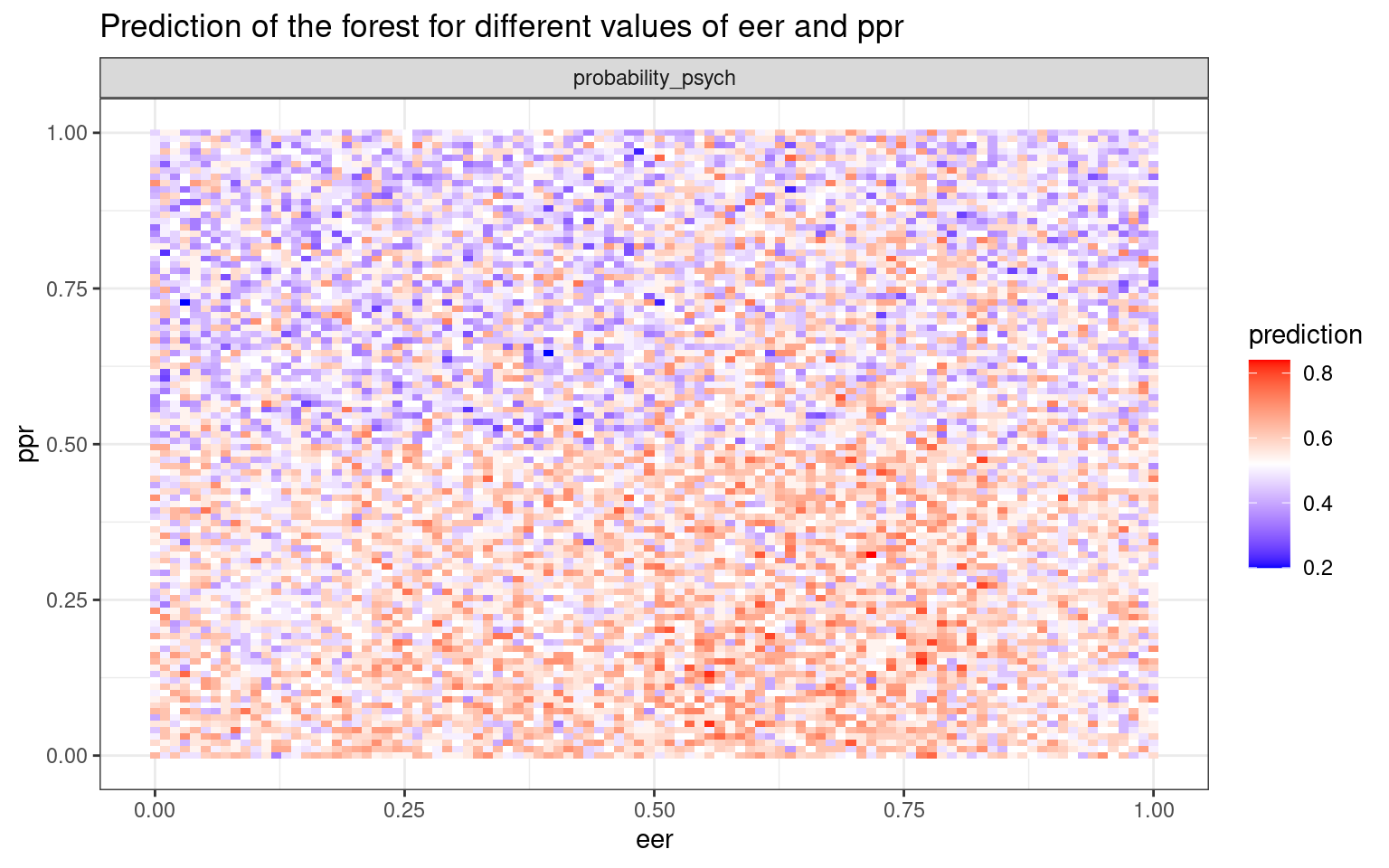phone <- read.csv("data_study1.csv")
phone.dt <- rpart(Smartphone ~ ., data = phone)
prp(phone.dt, cex = 0.5)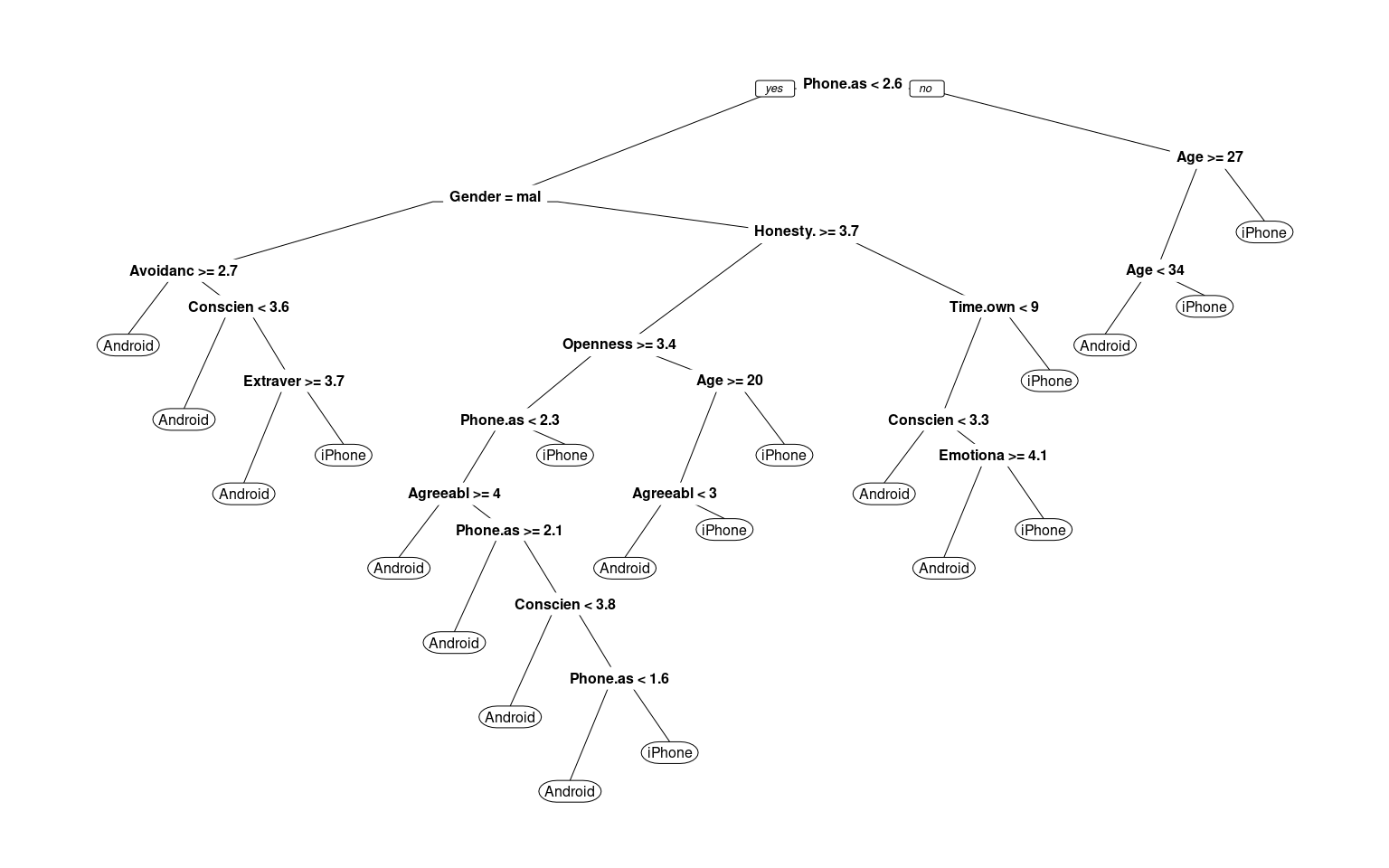confusion(phone$Smartphone, predict(phone.dt)[, 1] < 0.5) Overall accuracy = 0.783 Confusion matrix Predicted (cv) Actual [,1] [,2] [1,] 0.658 0.342 [2,] 0.129 0.871 ## this doesn't like the predicted value to be a factor phone$Smartphone<-
## phone$Smartphone=='iPhone' newer versions require this to be a factor: phone$Smartphone <- as.factor(phone$Smartphone) phone.rf <- randomForest(Smartphone ~ ., data = phone) phone.rf  Call: randomForest(formula = Smartphone ~ ., data = phone) Type of random forest: regression Number of trees: 500 No. of variables tried at each split: 4 Mean of squared residuals: 0.2234478 % Var explained: 7.9 confusion(phone$Smartphone, predict(phone.rf))
Overall accuracy = 0.002

Confusion matrix
Predicted (cv)
Actual  [,1]  [,2]  [,3]  [,4]  [,5]  [,6]  [,7]  [,8]  [,9] [,10] [,11] [,12]
Predicted (cv)
Actual [,13] [,14] [,15] [,16] [,17] [,18] [,19] [,20] [,21] [,22] [,23] [,24]
Predicted (cv)
Actual [,25] [,26] [,27] [,28] [,29] [,30] [,31] [,32] [,33] [,34] [,35] [,36]
Predicted (cv)
Actual [,37] [,38] [,39] [,40] [,41] [,42] [,43] [,44] [,45] [,46] [,47] [,48]
Predicted (cv)
Actual [,49] [,50] [,51] [,52] [,53] [,54] [,55] [,56] [,57] [,58] [,59] [,60]
Predicted (cv)
Actual [,61] [,62] [,63] [,64] [,65] [,66] [,67] [,68] [,69] [,70] [,71] [,72]
Predicted (cv)
Actual [,73] [,74] [,75] [,76] [,77] [,78] [,79] [,80] [,81] [,82] [,83] [,84]
Predicted (cv)
Actual [,85] [,86] [,87] [,88] [,89] [,90] [,91] [,92] [,93] [,94] [,95] [,96]
Predicted (cv)
Actual [,97] [,98] [,99] [,100] [,101] [,102] [,103] [,104] [,105] [,106]
Predicted (cv)
Actual [,107] [,108] [,109] [,110] [,111] [,112] [,113] [,114] [,115] [,116]
Predicted (cv)
Actual [,117] [,118] [,119] [,120] [,121] [,122] [,123] [,124] [,125] [,126]
Predicted (cv)
Actual [,127] [,128] [,129] [,130] [,131] [,132] [,133] [,134] [,135] [,136]
Predicted (cv)
Actual [,137] [,138] [,139] [,140] [,141] [,142] [,143] [,144] [,145] [,146]
Predicted (cv)
Actual [,147] [,148] [,149] [,150] [,151] [,152] [,153] [,154] [,155] [,156]
Predicted (cv)
Actual [,157] [,158] [,159] [,160] [,161] [,162] [,163] [,164] [,165] [,166]
Predicted (cv)
Actual [,167] [,168] [,169] [,170] [,171] [,172] [,173] [,174] [,175] [,176]
Predicted (cv)
Actual [,177] [,178] [,179] [,180] [,181] [,182] [,183] [,184] [,185] [,186]
Predicted (cv)
Actual [,187] [,188] [,189] [,190] [,191] [,192] [,193] [,194] [,195] [,196]
Predicted (cv)
Actual [,197] [,198] [,199] [,200] [,201] [,202] [,203] [,204] [,205] [,206]
Predicted (cv)
Actual [,207] [,208] [,209] [,210] [,211] [,212] [,213] [,214] [,215] [,216]
Predicted (cv)
Actual [,217] [,218] [,219] [,220] [,221] [,222] [,223] [,224] [,225] [,226]
Predicted (cv)
Actual [,227] [,228] [,229] [,230] [,231] [,232] [,233] [,234] [,235] [,236]
Predicted (cv)
Actual [,237] [,238] [,239] [,240] [,241] [,242] [,243] [,244] [,245] [,246]
Predicted (cv)
Actual [,247] [,248] [,249] [,250] [,251] [,252] [,253] [,254] [,255] [,256]
Predicted (cv)
Actual [,257] [,258] [,259] [,260] [,261] [,262] [,263] [,264] [,265] [,266]
Predicted (cv)
Actual [,267] [,268] [,269] [,270] [,271] [,272] [,273] [,274] [,275] [,276]
Predicted (cv)
Actual [,277] [,278] [,279] [,280] [,281] [,282] [,283] [,284] [,285] [,286]
Predicted (cv)
Actual [,287] [,288] [,289] [,290] [,291] [,292] [,293] [,294] [,295] [,296]
Predicted (cv)
Actual [,297] [,298] [,299] [,300] [,301] [,302] [,303] [,304] [,305] [,306]
Predicted (cv)
Actual [,307] [,308] [,309] [,310] [,311] [,312] [,313] [,314] [,315] [,316]
Predicted (cv)
Actual [,317] [,318] [,319] [,320] [,321] [,322] [,323] [,324] [,325] [,326]
Predicted (cv)
Actual [,327] [,328] [,329] [,330] [,331] [,332] [,333] [,334] [,335] [,336]
Predicted (cv)
Actual [,337] [,338] [,339] [,340] [,341] [,342] [,343] [,344] [,345] [,346]
Predicted (cv)
Actual [,347] [,348] [,349] [,350] [,351] [,352] [,353] [,354] [,355] [,356]
Predicted (cv)
Actual [,357] [,358] [,359] [,360] [,361] [,362] [,363] [,364] [,365] [,366]
Predicted (cv)
Actual [,367] [,368] [,369] [,370] [,371] [,372] [,373] [,374] [,375] [,376]
Predicted (cv)
Actual [,377] [,378] [,379] [,380] [,381] [,382] [,383] [,384] [,385] [,386]
Predicted (cv)
Actual [,387] [,388] [,389] [,390] [,391] [,392] [,393] [,394] [,395] [,396]
Predicted (cv)
Actual [,397] [,398] [,399] [,400] [,401] [,402] [,403] [,404] [,405] [,406]
Predicted (cv)
Actual [,407] [,408] [,409] [,410] [,411] [,412] [,413] [,414] [,415] [,416]
Predicted (cv)
Actual [,417] [,418] [,419] [,420] [,421] [,422] [,423] [,424] [,425] [,426]
Predicted (cv)
Actual [,427] [,428] [,429] [,430] [,431] [,432] [,433] [,434] [,435] [,436]
Predicted (cv)
Actual [,437] [,438] [,439] [,440] [,441] [,442] [,443] [,444] [,445] [,446]
Predicted (cv)
Actual [,447] [,448] [,449] [,450] [,451] [,452] [,453] [,454] [,455] [,456]
Predicted (cv)
Actual [,457] [,458] [,459] [,460] [,461] [,462] [,463] [,464] [,465] [,466]
Predicted (cv)
Actual [,467] [,468] [,469] [,470] [,471] [,472] [,473] [,474] [,475] [,476]
Predicted (cv)
Actual [,477] [,478] [,479] [,480] [,481] [,482] [,483] [,484] [,485] [,486]
Predicted (cv)
Actual [,487] [,488] [,489] [,490] [,491] [,492] [,493] [,494] [,495] [,496]
Predicted (cv)
Actual [,497] [,498] [,499] [,500] [,501] [,502] [,503] [,504] [,505] [,506]
Predicted (cv)
Actual [,507] [,508] [,509] [,510] [,511] [,512] [,513] [,514] [,515] [,516]
Predicted (cv)
Actual [,517] [,518] [,519] [,520] [,521] [,522] [,523] [,524] [,525] [,526]
Predicted (cv)
Actual [,527]
[ reached getOption("max.print") -- omitted 2 rows ]

This does not seem to be better than the other models for the iphone data, but at least is is comparable.

Note the ranger random forest gives maybe better results without

library(ranger)
r2 <- ranger(Smartphone ~ ., data = phone)
r2
Ranger result

Call:
ranger(Smartphone ~ ., data = phone)

Type:                             Classification
Number of trees:                  500
Sample size:                      529
Number of independent variables:  12
Mtry:                             3
Target node size:                 1
Variable importance mode:         none
Splitrule:                        gini
OOB prediction error:             36.11 % 
treeInfo(r2)
  nodeID leftChild rightChild splitvarID           splitvarName splitval
1      0         1          2          1                    Age    26.50
2      1         3          4          9 Phone.as.status.object     2.40
3      2         5          6          0                 Gender     1.50
4      3         7          8          6      Conscientiousness     4.65
5      4         9         10          8   Avoidance.Similarity     2.65
6      5        11         12          6      Conscientiousness     3.95
7      6        13         14          9 Phone.as.status.object     2.75
8      7        15         16          4           Extraversion     3.65
9      8        NA         NA         NA                   <NA>       NA
terminal prediction
1    FALSE       <NA>
2    FALSE       <NA>
3    FALSE       <NA>
4    FALSE       <NA>
5    FALSE       <NA>
6    FALSE       <NA>
7    FALSE       <NA>
8    FALSE       <NA>
9     TRUE    Android
[ reached 'max' / getOption("max.print") -- omitted 182 rows ]
confusion(phone$Smartphone, predictions(r2)) Overall accuracy = 0.639 Confusion matrix Predicted (cv) Actual Android iPhone Android 0.466 0.534 iPhone 0.239 0.761 # K Nearest-Neighbor classifiers Another non-model-based classifier are nearest-neighbor methods. These methods require you to develop a similarity or distance space–usually on the basis of a set of predictors or features. Once this distance space is defined, we determine the class by finding the nearest neighbor in that space, and using that label. Because this could be susceptible to noisy data, we usually find a set of $$k$$ neighbors and have them vote on the outcome. These are K-nearest neighbor classifiers, or KNN. ## Choosing K. The choice of $$k$$ can have a big impact on the results. If $$k$$ is too small, classification will be highly impacted by local neighborhood; if it is too big (i.e., if K = N), it will always just respond with the most likely response in the entire population. ## Distance Metric The distance metric used in KNN easily be chosen poorly. For example, if you want to decide on political party based on age, income level, and gender, you need to figure out how to weigh and combine these. By default, you might add differences along each dimension, which would combine something that differs on the scale of dozens with something that differs on the scale of thousands, and so the KNN model would essentially ignore gender and age. ## Normalization selection A typical approach would be to normalize all variables first. Then each variable has equal weight and range. ## Using KNN Unlike previous methods where we train a system based on data and then make classifications, for KNN the trained system is just the data. ## Libraries and functions. There are several options for knn classifers. Library: Class Function: knn knn.cv ### Library: kknn Function: kknn This provides a ‘weighted k-nearest neighbor classifier’. Here, weighting is a weighting kernel, which gives greater weights to the nearer neighbors. ### Library: klaR Function: sknn A ‘simple’ knn function. Permits using a weighting kernel as well. ### Function: nm This provides a ‘nearest mean’ classifier ## Example In general, we need to normalize the variables first. phone <- read.csv("data_study1.csv") # Normalizing the values by creating a new function normal = function(x) { xn = (x - min(x))/(max(x) - min(x)) return(xn) } phone2 <- phone phone2$Gender <- as.numeric(as.factor(phone2$Gender)) for (i in 2:ncol(phone2)) { phone2[, i] = normal(as.numeric(phone2[, i])) } # checking the range of values after normalization par(mfrow = c(2, 1)) boxplot(phone[, 3:12]) boxplot(phone2[, 3:12])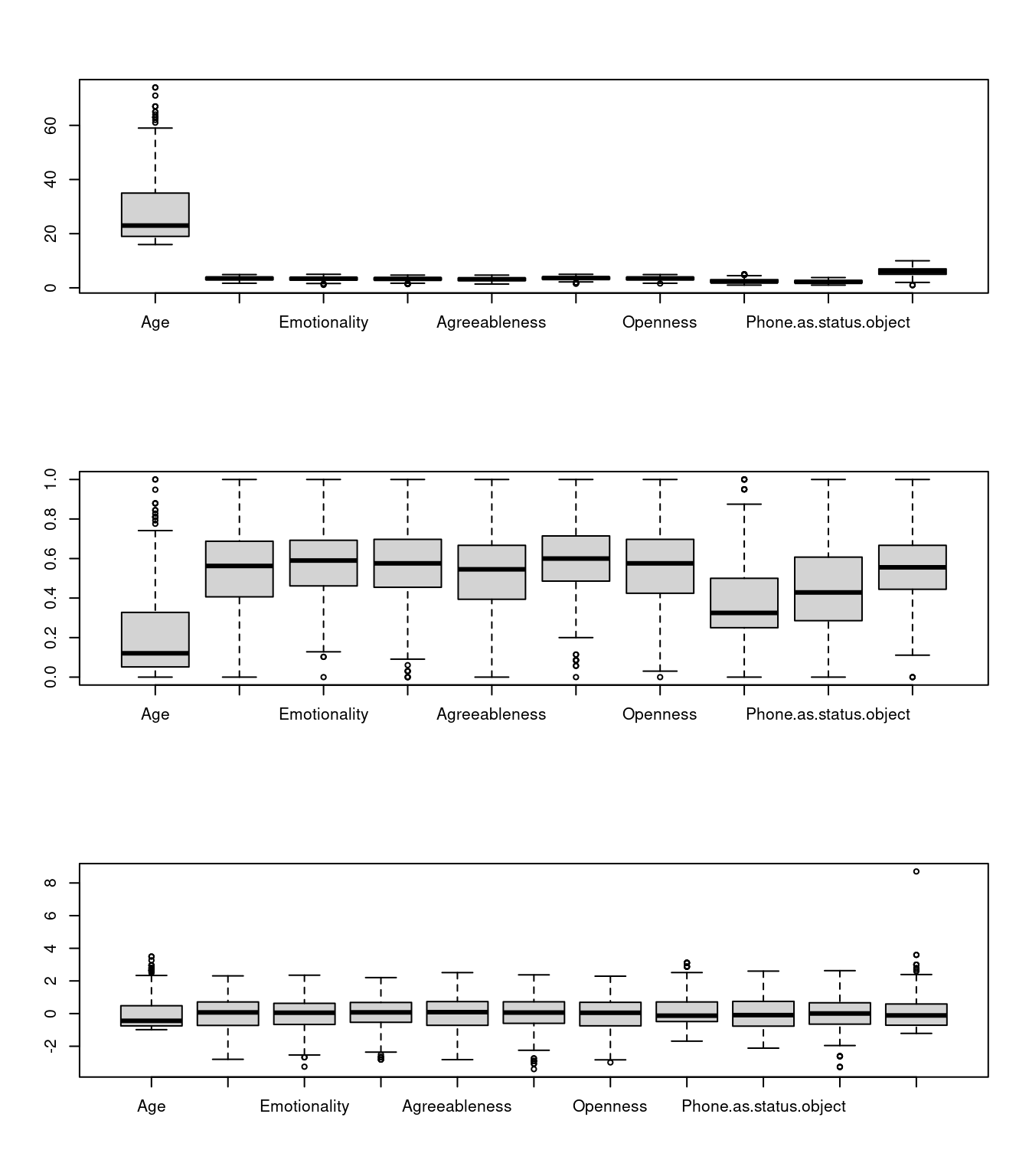## fitting a simple model library(class) m1 = knn(train = phone2[, -1], test = phone2[, -1], cl = phone2$Smartphone, k = 10)
confusion(phone2$Smartphone, m1) Overall accuracy = 0.707 Confusion matrix Predicted (cv) Actual [,1] [,2] [1,] 0.584 0.416 [2,] 0.206 0.794 That is pretty good, but what if we expand k m1 = knn(train = phone2[, -1], test = phone2[, -1], cl = phone2$Smartphone, k = 25)
confusion(phone2\$Smartphone, m1)
Overall accuracy = 0.686

Confusion matrix
Predicted (cv)
Actual  [,1]  [,2]
[1,] 0.534 0.466
[2,] 0.206 0.794

It looks we the choice of k is not going to matter much. What we could do is play with cross-validation though–right now we are testing on the same set as we are ‘training’ with, which will boost our accuracy (since the correct answer will always be one of the elements.) We can also try to do variable selection to change the similarity space to something that works better.

phone2 <- phone2[order(runif(nrow(phone2))), ]  #random sort
phone.train = phone2[1:450, 2:12]
phone.test = phone2[451:nrow(phone2), 2:12]
phone.train.target = phone2[1:450, 1]
phone.test.target = phone2[451:nrow(phone2), 1]

train <- sample(1:nrow(phone2), 300)
m2 <- knn(train = phone.train, test = phone.test, cl = phone.train.target, k = 10)
confusion(phone.test.target, m2)
Overall accuracy = 0.608

Confusion matrix
Predicted (cv)
Actual  [,1]  [,2]
[1,] 0.613 0.387
[2,] 0.396 0.604`